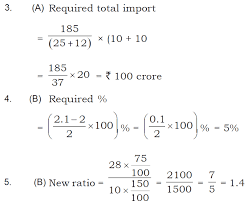# Data Interpretation Practice Questions for Bank Exams, RBI Assistants

### Data Interpretation Practice Questions for Bank Exams, RBI Assistants

Direction (1 – 5): Study the following bar graph and answer the given questions.
Following bar graph shows the ratio between export and import of two companies P and Q over given years 2011 to 2016.1. In which of the given years was the difference between the export and the import of company P is maximum?
a) 2012
b) 2015
c) 2014
d) 2016
e) 2013
2. In how many years the export is more than twice the import of company Q?
a) One
b) Two
c) Three
d) Four
e) Five
3. If the total exports of company Q for the years 2012 and 2016 was Rs.185 crore, then what was its total import for these two years? (in crore)
a) Rs.100
b) Rs.125
c) Rs.165
d) Rs.180
e) Cannot be determined
4. Ratio of export and import of company P in 2014 is how many percentage more/less than ratio of export and import of company Q in year 2013?
a) 1%
b) 5%
c) 10%
d) 20%
e) Cannot be determined
5. If the import of company Q is increased by 50% and export is decreased by 25% in year 2014, then what will be the new ratio between export and import for company Q in that year?
a) 1.2
b) 1.4
c) 1.5
d) 2.1
e) 310
Direction (6 – 10): Read the line graph carefully and answer the given question :
6. What is the total number of students hired by Jet for all city?
a) 2150
b) 2350
c) 2450
d) 3000
e) None of these
7. What is the total number of student hired by all the companies for Patna city?
a) 2500
b) 2400
c) 2450
d) 2300
e) None of these
8. What is the difference between number of students hired by all the companies for mumbai city and patna city?
a) 950
b) 850
c) 900
d) 925
e) None of these
9. What isthe average number of students hired by Indigo for all cities taken together?
a) 530
b) 630
c) 430
d) 420
e) None of these
10. What is the difference between total number of students and average number of students hired by all companies for Kolkata city?
a) 1825
b) 1925
c) 1725
d) 2025
e) None of these
Solutions:
1. E)
2. C)6. A) Required total no. of students = 450 + 200 + 500 + 350 = 650 = 2150
7. D) Required total no. of students = 700 + 650 + 600 + 350 = 2300
8. C) Total no. of students hired for Mumbai city = 500 + 400 + 300 + 200 = 1400
Total no. of students hired for Patna city = 700 + 650 + 600 + 350 = 2300
Therefore, required difference = 2300 – 1400 = 900
9. E) Required Average = (350 + 300 + 400 + 700 + 300)/5 = 2050/5 = 410
10. E) Total no. of student hired by all companies for Kolkata city = 650 + 550 + 450 + 300 = 1950 and its average = 1950/5 = 390
Therefore, required difference = 1950 – 390 = 1560